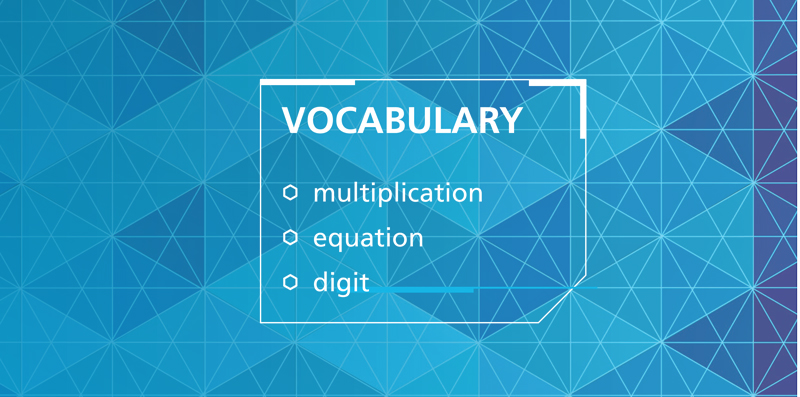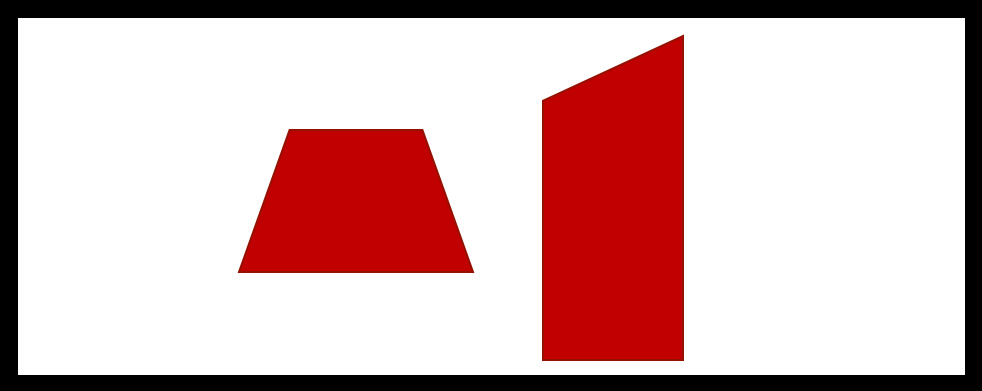Select Page## Q&A: Mathematical Vocabulary in Investigations 3

Question: How does Investigations approach the teaching and learning of mathematical vocabulary?Answer: In Investigations 3, mathematical vocabulary is introduced as a natural part of the conversation during activities and discussions, along-side students’ own words....## Q&A: The Definition of a Trapezoid

Question: Why did you decide to use the exclusive definition of a trapezoid?Answer: As the question suggests, there is more than one definition of a trapezoid. Mathematicians define trapezoids in one of two ways:Using the inclusive definition, all parallelograms...